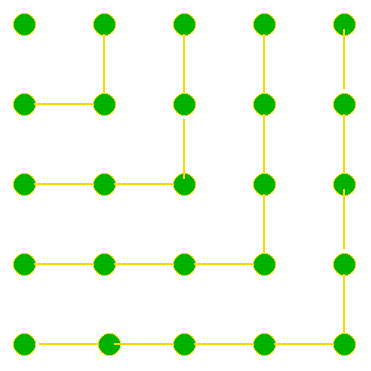#### You may also like### Triangular Triples

Show that 8778, 10296 and 13530 are three triangular numbers and that they form a Pythagorean triple.### IffThe diagram illustrates the formula: 1 + 3 + 5 + ... + (2n - 1) = n ² Use the diagram to show that any odd number is the difference of two squares. Note that 15 = 8 ² - 7 ² as well as 4 ² - 1 ². Write the number 105 as the difference of two squares in as many different ways as you can? The number 1155 can be written as the difference of two squares in eight different ways, can you find them?